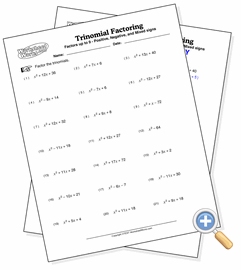# Trinomial Factoring

## No Variable CoefficientsDevelop basic trinomial factoring skills

These are basic trinomial factoring problems where the second degree term has no coefficient. The task, then, is to find the distribution of the factors which will add up to the middle coefficient of each trinomial.